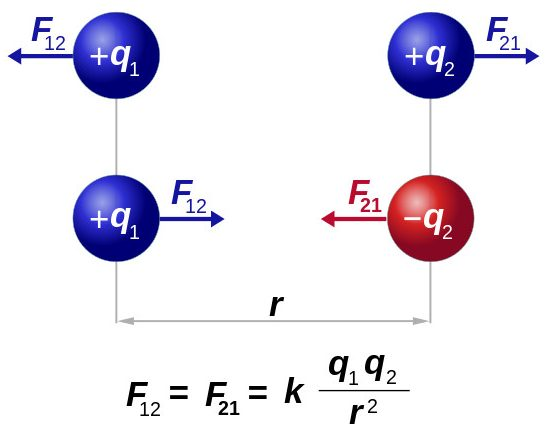Science Forums

# Equation For Strong Nuclear Force And Weak Nuclear Force

## Recommended Posts

Hello, I was wondering if there is equation for the Strong Nuclear Force Strength and Weak Nuclear Force Strength like Coulomb's Law or Newtonian Gravity. I would like it in Function of Force Form if possible. F(x) = (x) , It seems as if most of the other forces have equation such as this that explain it.

Newtonian Law of GravityCoulomb's Law of Electric ForceLorentz Law of Magnetism

Edited by Vmedvil
##### Share on other sites

Hello, I was wondering if there is equation for the Strong Nuclear Force Strength and Weak Nuclear Force Strength like Coulomb's Law or Newtonian Gravity. I would like it in Function of Force Form if possible. F(x) = (x) , It seems as if most of the other forces have equation such as this that explain it.

Newtonian Law of GravityCoulomb's Law of Electric Force

Lorentz Law of Magnetism

This is not my field, but there is an interesting discussion of this question here: https://physics.stackexchange.com/questions/8452/is-there-an-equation-for-the-strong-nuclear-force

It does not seem as straight forward as electromagnetism or gravitation.

##### Share on other sites

This is not my field, but there is an interesting discussion of this question here: https://physics.stackexchange.com/questions/8452/is-there-an-equation-for-the-strong-nuclear-force

It does not seem as straight forward as electromagnetism or gravitation.

That is very helpful that is in the form that I am seeking almost, Now what about the WNF. This though not as straight forward as EM or G is still much closer than any that I have found even though it is particle specific, meaning different for all particle pairs. The second equation in this page is much more general, based on the Yukama potential, add a 1/ Δx and it is Force, Now what about the WNF?

Edited by Vmedvil
##### Share on other sites

That is very helpful that is in the form that I am seeking almost, Now what about the WNF. This though not as straight forward as EM or G is still much closer than any that I have found even though it is particle specific, meaning different for all particle pairs. The second equation in this page is much more general, based on the Yukama potential, add a 1/ Delta Time and Mass and it is in force. Now what about the WNF?

I found the link by a google search. Why don't you now do that for the weak force and show us what you find?

##### Share on other sites

I found the link by a google search. Why don't you now do that for the weak force and show us what you find?

Lol, you googled and found that huh? Well, I googled and found this long equation for the WNF, I dunno I think you are better at this than I am.

##### Share on other sites

Lol, you googled and found that huh? Well, I googled and found this long equation for the WNF, I dunno I think you are better at this than I am.

Yes I've now had a look too, and see what you mean. I can't help you further I'm afraid.

## Join the conversation

You can post now and register later. If you have an account, sign in now to post with your account.×   Pasted as rich text.   Paste as plain text instead

Only 75 emoji are allowed.

×   Your previous content has been restored.   Clear editor

×   You cannot paste images directly. Upload or insert images from URL.